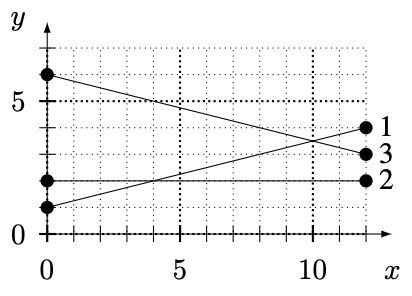시간 제한 메모리 제한 제출 정답 맞은 사람 정답 비율
15 초 512 MB 0 0 0 0.000%

## 문제

A new era of aviation is upon us - the first solar-powered jumbo jets are about to be made available for public travel! However, this cutting-edge technology raises some safety concerns, as the rays of sunlight which power these planes can be blocked by other objects in the sky. As such, some statistics must first be calculated concerning the planned initial flights.

We consider a set of N flight paths, all travelling East from one city to another. A plane can be considered as a single point. The sky through which they pass can be modelled as a Cartesian plane, with x-coordinates representing distance East from an arbitrary fixed point, and y-coordinates representing altitude. We are interested only in the section of the sky with x-coordinates in the inclusive range [0, X] (1 ≤ X ≤ 109), in which all flight paths are straight lines. The ith plane flies from (0, Ai) to (X, Bi) (1 ≤ Ai, Bi ≤ 109). All A values are distinct, as are all B values. The planes travel at unknown, possibly non-constant speeds along their flight paths, so at any point in time, a plane may be anywhere along its path. However, it is known that the planes will never crash with one another, so if two flight paths cross one another, both planes won’t reach the intersection point at precisely the same time.

Planes also have an inteference factor: each plane i has an interference factor Ci (1 ≤ Ci ≤ 109), which is a measure of how much plane i would negatively impact the solar absorbing capability of any plane below them.

The solar panels on each plane are rather strange, in that they can only collect energy from directly above the plane. This means the sunlight that a given plane can absorb can be obstructed by other planes which occupy the same x-coordinate as it, and have a larger y-coordinate than it. In particular, their effectiveness is reduced based on the sum of the interference factor of all such planes.

Given this information, as well as a fixed distance constant K (1 ≤ K ≤ X), you must answer Q queries regarding the possible impact on planes’ solar panels at various times. The ith query asks for the largest possible amount by which the plane Pi’s solar panels could be obstructed at a single moment in time, at any point while the plane’s x-coordinate is in the inclusive range [Si, Si + K] (0 ≤ Si ≤ X − K).

## 입력

The first line contains four space-separated integers: X (1 ≤ X ≤ 109), the maximum x-coordinate to consider; K (1 ≤ K ≤ X), the fixed distance constant; N (1 ≤ N ≤ 2000), the number of flight paths; Q (1 ≤ Q ≤ 800 000), the number of queries.

Each of the next N lines contain three integers, Ai, Bi, and Ci, for i = 1..N (1 ≤ Ai, Bi, Ci ≤ 109), representing, for plane i, the starting y-coordinate, the ending y-coordinate, and the interference factor (respectively).

Each of the next Q lines contain two integers, Pi and Si, for i = 1..Q representing the query concerning plane Pi while its x-coordinate is in the range [Si, Si + K].

## 출력

The output consists of Q lines, each with the integer answer to the ith query, for i = 1..Q.

## 예제 입력 1

12 4 3 3
1 4 5
2 2 3
6 3 6
2 1
1 8
3 0


## 예제 출력 1

11
6
0


Below is a diagram of the planes’ flight paths:The first query is for plane 2 over x-coordinates in the inclusive range [1, 5]. While this plane is at an xcoordinate smaller than or equal to 4, it might be covered by plane 3, but definitely not by plane 1. However, when it’s at an x-coordinate larger than 4, it might be covered by both other planes. Therefore, the answer to this query is the sum of the other planes’ interference factors, 5 + 6 = 11.

For the second query, plane 1 could be covered by plane 3 while it has x-coordinate less than 10, and will not be covered by anything while it has x-coordinate greater than or equal to 10. Thus, it is only possibly interfered with by plane 3 with interference factor 6.

Neither plane 1 nor plane 2 can possibly be directly above plane 3 until it reaches x-coordinate 10, so the answer to the final query is 0.